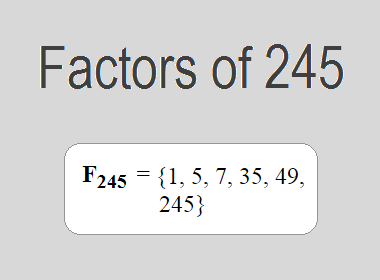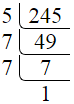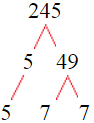# Factors of 245The factors of 245 are 1, 5, 7, 35, 49, and 245 i.e. F245 = {1, 5, 7, 35, 49, 245}. The factors of 245 are all the numbers that can divide 245 without leaving a remainder.

We can check if these numbers are factors of 245 by dividing 245 by each of them. If the result is a whole number, then the number is a factor of 245. Let's do this for each of the numbers listed above:

·        1 is a factor of 245 because 245 divided by 1 is 245.

·        5 is a factor of 245 because 245 divided by 5 is 49.

·        7 is a factor of 245 because 245 divided by 7 is 35.

·        35 is a factor of 245 because 245 divided by 35 is 7.

·        49 is a factor of 245 because 245 divided by 49 is 5.

·        245 is a factor of 245 because 245 divided by 245 is 1.

## How to Find Factors of 245?

1 and the number itself are the factors of every number. So, 1 and 245 are two factors of 245. To find the other factors of 245, we can start by dividing 245 by the numbers between 1 and 245. If we divide 245 by 2, we get a remainder of 1. Therefore, 2 is not a factor of 245. If we divide 245 by 3, we get a remainder of 2. Therefore, 3 is not a factor of 245.

Next, we can check if 4 is a factor of 245. If we divide 245 by 4, we get a remainder of 1. Therefore, 4 is not a factor of 245. If we divide 245 by 5, we get a remainder of 0. Therefore, 5 is a factor of 245. We can continue this process for all the possible factors of 245.

Through this process, we can find that the factors of 245 are 1, 5, 7, 35, 49, and 245. These are the only numbers that can divide 245 without leaving a remainder.

********************

********************

## Properties of the Factors of 245

The factors of 245 have some interesting properties. One of the properties is that the sum of the factors of 245 is equal to 342. We can see this by adding all the factors of 245 together:

1 + 5 + 7 + 35 + 49 + 245 = 342

Another property of the factors of 245 is that they are all odd numbers. This is because 245 is an odd number, and any even number cannot divide an odd number without leaving a remainder.

Another property of the factors of 245 is that the prime factors of 245 are 5 and 7 only.

## Applications of the Factors of 245

The factors of 245 have several applications in mathematics. One of the applications is in finding the highest common factor (HCF) of two or more numbers. The HCF is the largest factor that two or more numbers have in common. For example, to find the HCF of 245 and 35, we need to find the factors of both numbers and identify the largest factor they have in common. The factors of 245 are 1, 5, 7, 35, 49, and 245. The factors of 35 are 1, 5, 7, and 35. The largest factor that they have in common is 35. Therefore, the HCF of 245 and 35 is 35.

Another application of the factors of 245 is in prime factorization. Prime factorization is the process of expressing a number as the product of its prime factors. The prime factors of 245 are 5 and 7 since these are the only prime numbers that can divide 245 without leaving a remainder. Therefore, we can express 245 as:

245 = 5 × 7 × 7

We can do prime factorization by division and factor tree method also. Here is the prime factorization of 245 by division method,245 = 5 × 7 × 7

Here is the prime factorization of 245 by the factor tree method,245 = 5 × 7 × 7

## Conclusion

The factors of 245 are the numbers that can divide 245 without leaving a remainder. The factors of 245 are 1, 5, 7, 35, 49, and 245. The factors of 245 have some interesting properties, such as being odd numbers and having a sum of 342. The factors of 245 have several applications in mathematics, such as finding the highest common factor and prime factorization.